## //----------------------------// ArtMatic 2 in 2 out components //----------------------------//

Introduction
Maths tools #
Scale Ax, By
Scale & Offset
Rotate
Skew
Normalise
Edge Logic #
Undulations
Waves
Sin warp
Sin x, sin y
Hexagonal Sin
Orthogonal Tiles #
Orthogonal Mirror Tiles
Other Tiles #
Mirrors N Tiles #
Pixel prism
Crystallize
Disk Tiling
Sierpensky tiles
Ripples
Split & Move
infinity disk gate
Mirror
Mirrors 3
Mirrors 4
N-Fold Mirrors #
Branch N
Disk Mirror
Distance Mirror
Disk Inverse Ring
Complex inverse
Complex Moebius
Complex Map
Complex Map 2
Complex waves
Ring Space
Polar Space
Orbiters
Twirl
Multi twirls
Tech displace
Tech quantize
Random noise
Low Freq Random
Perlin random
Multi Perlin displace
2D turbulence
Time turbulence
MultiFractal displace
Ridged Fractal displace
Chaos displace A
Chaos displace B
Crystal displace
Random Fractal Space
Spots displace
Directional Random
Bubble Space
Facet space
Facet space mirrors
Facet space rotate
22 Compiled tree

## Introduction

These functions maps 2D input to a 2D output and are mostly space transformations (mirroring, rotations, tiling etc) or distortions (displacements, complex transforms, warpings etc). Transforming space on top of the tree will alter the geometry of any components below. Some like "22 edge logic" are actually more like 2 combined 21 tiles, one that outputs one type of values in X and another in Y.
Some of these components can also act as two parallel 1D filters.
A good way to see what effect these functions have on a system is to use a basic structure where a 2D Vector function distorts a picture.

An essential in-depth discussion of ArtMatic structures Trees and components is found in ArtMatic Designer References and in Building trees.

##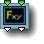22 Maths tools #

parameters :
A : Scale (-25. : 25.)
B : Rotation (-360. : 360.)
C : Offset x (-25. : 25.)
D : Offset y (-25. : 25.)

discussion :
Many useful 2D space transforms are provided as various algorithm in the 2D Maths tools tile.

algorithms :
• Affine (scale+rotate+translate) :
Combine scaling, rotation and offset in a single transform. Options allows to control the order of transformations.
Options:
-Use post translate
-Use post translate*scale
Translation applied after the rotation + scale. The offset is interpreted in transformed space orientation.
-Use pre translate
-Use pre translate/scale
Translation applied before the rotation + scale. The offset is interpreted in original space orientation, scaled when using pre-translate and unscaled when using pre translate/scale.
When there is no rotation "Use pre translate/scale" is equivalent to post translate. With rotation the resulting motion can be quite different if translation is applied before or after the transform. When you want the translated position be the center of rotation use the pre-translate modes.
• Affine inverse :
Does the inverse transform if you set it with the same parameters as the above.
• Affine exp :
Like "Affine", Affine exp combine scaling, rotation and offset in 1 transform but the scaling is using exponentials. Scaling is set by exp('Scale') meaning 'Scale' at zero (exp(0)=1) is identity. 'Scale' goes from -8 to 8 which makes a zoom range of approximatively 1/2980x to 2980x.
This transform, added in ArtMatic Engine 8.0, is particularly suited to animate objects and to zoom in fractals images. Affine exp has the same options as Affinep transform. When using it to zoom inside fractals use the "post translate" mode so that zoom and rotations are centered whatever the translations.
Example file : Libraries/Components demo/Affine Exponential.artm,
Libraries/Components demo/Mandelbrot Exp zoom.artm

• Polar Log sym :
Return a polar logarithmic space with x out as atan(ABS(x),y) and y out as log(x^2+y^2)
• Polar Log :
Return a polar logarithmic space with x out as atan(x/y) and y out as log(x^2+y^2). Polar Log sym and Polar Log transforms circles into circles. Lines in y are transformed into circles. When you rotate the resulting space you will obtain partial spirals. When space is tiled there are some precise combinaison of scaling and rotation that can yield perfect spirals when tiles of another level connects exactly. Log spirals below makes finding these values easier for tiling that have whole number period.
• Log Spirals :
Return a polar logarithmic space scaled and rotated to create spirals that can match various tiling as set by the optional menu. The combination of 'Branch' and 'jump' parameters creates the scaling and rotation suited to have a joining spiral given the particular tiling structure (square, triangle, hexagonal..) set by the options. They should be kept whole numbers most of the time. See various examples in the Libraries/Maths/ folder.

• Complex z2 :
Return Z^2+ (C+iD) in the complex plane. Parameters C & D sets the offset and the seed of the julia set when the transform is applied iteratively.
• Complex z3 :
Return Z^3+ (C+iD) in the complex plane. Parameters C & D sets the offset and the seed of the julia set when the transform is applied iteratively.

• Complex Cz^N (8.08 addition) :
Return C * Z^N in the complex plane. N (integer) is ranging 2 to 12 and is set with third parameter; Parameters C real and C imaginary sacales the result. Keep C at (1,0) for a straight complex power.

• Complex inverse (1/Az) :(fixed misleading name in 8.08)
Return the complex inverse 1/A(x+iy) without translation and with A set by parameters. Keep A at (1,0) for a straight complex inversion. Like Complex Log and Moebius transforms, Complex inverse transforms circles into circles.
• Complex inverse (C/z) (8.08 addition) :
Return the complex inverse C/(x+iy) with C given by parameters C real and C imaginary.

• Complex z-(1./z) :
Subtract the complex inverse from z(x+iy).
• Complex Exp :
Returns the complex exponential. The complex exponential function is given exp(Z) =e^x cos(y)+ i e^x + i sin(y) with Z=x+iy.
• Complex Log :
Returns the complex logarithm, the inverse of exponential. Complex Log is defined by 0.5*log(x^2+y^2)) + i*atan(x/y). Complex Log is similar to Polar Log but with x & y exchanged (or Z rotated 90 degree). It transforms circles and lines in x into circles.
• Complex Sines :
• Complex Hyperbolic Sines :
Returns the complex hyperbolic sines given by sinh(x) * cos(y)+ i cosh(x) * sin(y).
• Moebius BC :
Moebius transform Az+B / Cz+D here with A at 1 and B & C set by parameters. D is derived to satisfy AD-BC==1
• Moebius BC inverse :
Returns the inverse of Moebius BC with the same parameters.
• Moebius parabolic :
One fixed point Moebius transform. The parabolic property means also that the trace of the A B C D matrix is +- 2
• Moebius 2 fixed points :
A 2 fixed point Moebius transform. Parameter A and B set the K factor. When (1,0) the space is untransformed. When the real part of K is negative space is contained in the unit sphere as shown in the example file at Libraries/Maths/Complex maths/2fixed point moebius K factor. Parameter CD sets one fixed point, the other taken in mirror as(-C,D).

• Abs + offset :
Returns the absolute value of x and y translated by 'Offset x' & 'Offset y'. It is similar to the Mirrors 4 tile but without the scaling.
• |x|- sign(x)*y :
Without parameters this function returns a 180 degrees rotation of positive x. Resulting space is discontinuous around x=0, but symmetrical by a 180 rotation.
• |x|+ sign(x)*y :
Without parameters this function returns a 180 degrees rotation of negative x. Resulting space is discontinuous around x=0, but symmetrical by a 180 rotation.

##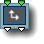22 Scale Ax, By

parameters :
A : Scale x (-8. : 8.)
B : Scale y (-8. : 8.)

discussion :
This simple primitive scales the two inputs independently. The scale parameters have a greater range than the similar Scale and Offset function. Note that the x output is solely a function off the x input, and the y output is solely a function of the y input, Hence, this component can be used as either a space transform function OR as two parallel 1D filters. When used as a space transform, this component acts as a magnification function.

Left output : A * x
Right output: B * y

##22 Scale & Offset

parameters :
A : Scale (-16. : 16.)
B : Offset x (-32. : 32.)
C : Offset y (-32. : 32.)

##22 Rotate

parameters :
A : Angle (-180. : 180.)
B : Center x (-32. : 32.)
C : Center y (-32. : 32.)

##22 Skew

parameters :
A : Skew Angle (-3.14 : 3.14)
B : Skew Amount (-2. : 2.)
C : Scale (-4. : 4.)

discussion :
Skew skews the incoming space. The 'Skew Angle' parameter determines the angle of the skewing. 'Skew Amount' influences the amount of skewing, and 'Scale' zooms the space.algorithms :
• Default skew :
• Skew Lateral :
This skewing ensures that the y is invariant.
• Skew Vertical :
This skewing ensures that the x is invariant.

##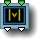22 Normalise

parameters :
A : Magnitude (0.50 : 8.)

discussion :
This is a mathematical primitive that normalizes the incoming 2D vector(x,y) by dividing it with its length (which sets it to 1). Normalisation is mandatory when computing dot products for illumination but it can be used also to create non linear mappings and space distortions effects.

##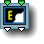22 Edge Logic #

parameters :
A : Offset y (-10. : 10.)
B : Edge height (0. : 10.)
C : Edge width (0. : 10.)
D : Smoothness % (0. : 10.)

discussion :
Various logic tools have the option to create an edge at the intersection in boolean operations. But it is often useful to get the edge independently so they can be shaded separately. The 22 Edge Logic tool sends the edge distance field (DF) on the first output and the boolean operation on the second. This order is consistent with other 3D objects that sends 2 outputs like
DF Polyhedrons #, Buildings, DF Constructs # and DF Cities #. The 42 "logic tools" introduced in AM 7. 0 can be used to mix various 2 DF Edge logic outputs.

Parameters :

• Offset y : Is added to input y prior the boolean operation.
• Edge height & Edge width : Sets the global edge width & height. For very large or very small object the range may be too small then you can uses the dynamic option menu to interpret the parameters as *10 or /10.
• Smoothness : As usual to smooth the resulting volume. Don't set it too high or the edge will disappear.
algorithms :
• Edge Union :
Union input A with B and outputs the edge in x and the union in y
• Edge Intersect :
Intersect input A with B and outputs the edge in x and the intersection in y
• Edge Subtract :
Subtract input B from A and outputs the edge in x and the result volume in y

• Edge Subtract Example.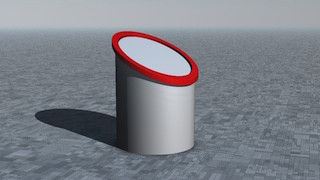• Chisel Edge Union :
Union of xy -> out2 with Chisel edge to out1
• Chisel Edge Intersect :
Intersect of xy -> out2 with Chisel edge to out1
• Chisel Edge Subtract :
Subtract (x-y) -> out2 with Chisel edge to out1

• Chisel Edge Subtract Example.
Here the volume has been shrunk to better see the generated "chiseled" edges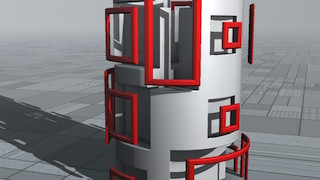• Plane Subtract :
Instead of an edge Plane subtract outputs in out 1 the DF of a plane that follows the shape of the subtracted DF. (It will be a curved plane if the subtracted DF is a sphere for example).
• Plane Intersect :
• Edge Partial Subtract :
The first parameter is the subtract width. It subtracts partially B to A and returns the edge in out1 and the main volume in out2.
• Edge Partial Subtract Example.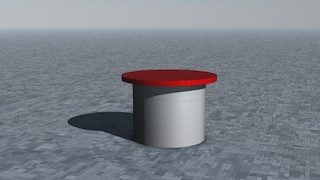• Echoes Union :
• Echoes Intersect :
• Echoes Subtract :

• The main logic functions with 3 edge to out1. The offset between edges is the first parameter. When negative the edges are following the x volume and the y volume on positive.

##22 Undulations (former x+Asin y, y+Asin x)

parameters :
A : Amplitude x (-4. : 4.)
B : Amplitude y (-4. : 4.)
C : Frequency (-32. : 32.)
D : Phase (-32. : 32.)

discussion :
22 Undulations displace space using sine waves, thus "undulating" the coordinates. When the 'Amplitude' is 0, space is not distorted. In 8.08 amplitudes can be set for X and Y axis separately. A great variety of space distortions and patterns can be generated by adjusting the amplitudes and frequency. In 8.08 the phase parameter allow to animates the undulations and the Frequency parameter has the usual
frequency options settings. In DF modes the frequency adjust the amplitudes to make the transform compatible with DF modeling. Low frequency DF undulation can be used for example to curve a DF city for 3D rendering in ArtMatic Voyager.##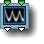22 Waves

parameters :
A : Frequency (-2. : 2.)
B : Amplitude x (-2. : 2.)
C : Amplitude y (-2. : 2.)

discussion :
This component bends space giving it a "waved" aspect. The distortion is created using the absolute value of the sine function. 'Frequency' determines the spacing of the undulations. The amplitude parameters determine the amount of horizontal and distortion.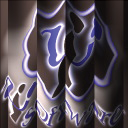##22 Sin warp

parameters :
A : Amplitude (-6. : 6.)
B : Frequency (-6.28 : 6.28)

discussion :
This component is very similar to the x*A sin y/y*A sin x component and bends space with waves or an eggcrate-like effect.

##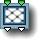22 Sin x, sin y

parameters :
A : Amplitude (-8. : 8.)
B : Frequency (0. : 16.)

discussion :
This component tiles space by applying the sine function to the incoming values. The function's output always falls in the range of -1 to 1. This is a great way to create tiles and other repetitive patterns and textures.

The output of this function is both periodic and continuous, thus it is often used for sound design.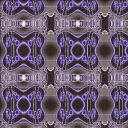##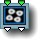22 Hexagonal Sin

parameters :
A : Scale (0.50 : 8.)

discussion :
Like
Sin x, sin y, this component tiles space. In this case, space is tiled using a combination of sin and hexagonal transforms. The output of this function is both periodic and continuous, thus it is often used for sound design.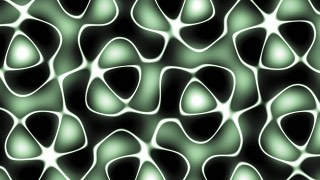A plane and a rotating line passed through the Hexagonal Sin space.

##22 Orthogonal Tiles #

parameters :
A : Scale (0. : 16.)
C : Frequency (0. : 8.)

discussion :
Orthogonal Tiles # provides several methods for tiling space. The parameters are algorithm dependent. 'Frequency' sets the tile size and leaves the coordinates of each tile unchanged and centered at 0. The 'Scale' parameter scales the space in the tiles with 1 being no change. The rectangle algorithms allows independent setting of the x and y tile frequency.

Tip: To create complex symmetry, follow this component with the Mirrors 4 component.

Warning: The output is highly discontinuous and is to be avoided in systems destined for ArtMatic Voyager.

algorithms :
• Unit Square Tiles :
Square tiling on the unit grid when frequency is at 1.
• Square Tiles 45 degrees :
45° square tiling scaled by square root of 2 so that edges passes through the unit grid.
• Rectangle Tiles :
Rectangular tiling ; "frequency x" and "frequency y" can be adjusted independently.
• Square Tiles displaced ,
• Zoom Tiles/Pi displaced (old) :
Theses algorithms feature a displacement parameter which creates non-repetitive tiling by applying random displacements for the tiles. When 'displacement' is non-zero, the tiling is non-repetitive.

##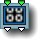22 Orthogonal Mirror Tiles

parameters :
A : Scale (0. : 32.)
B : Aspect ratio (0. : 2.)

discussion :
The input space is mirrored both vertically and horizontally and then tiled which makes it ideal for creating tiled wallpaper or kaleidoscope type effects. (For creating individual tiles for export, the Mirrors 4 component may be more appropriate.) Parameter 'Scale' is applied both horizontally and vertically. Parameter 'Aspect ratio' applies only vertically and can be used to achieve rectangular mirrors tilings.

##22 Other Tiles #

parameters :
A : Amplitude (0. : 8.)
B : Rotation (-180. : 180.)
C : Frequency (0. : 8.)

discussion :
This component provides a collection of periodic regular tilings. 'Amplitude' scales the space in each tile while 'Rotation' rotates it. 'Frequency' sets the period of the tiling.

algorithms :
• Triangle Tiling :
Tile orientation is constant.
• Triangle Tiling Alt :
Tile orientation is reversed alternatively.
• Triangle Tiling 6 :
Tile orientation is using a sixfold rotation.
• Lozenges Tiling :
3 lozenges makes an hexagon when tiles are rotated 120°.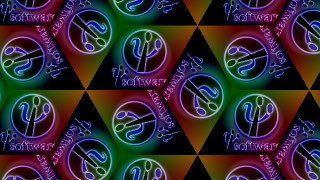• Lozenges mirror Tiling :
• Hexagonal Tiling :
• Hexagonal Zoom Tiling :
• Triangles 45 degrees :
A square lattice split at 45° where the tile orientation alternates.

• Cairo 18 degrees Tiling,
• Cairo 30 degrees Tiling :

•##22 Mirrors N Tiles #

parameters :
A : Tile size (0.12 : 50.)
B : Phase (0. : 8.)

discussion :
Mirrors N Tiles implements a series of tiling with mirror symmetries around the edges. The tilings are periodic and many uses N rotational foldings. The 'Phase' parameters moves the Y coordinate in the given orientation options. "Tile size" sets the period of the tiling. The "60 degrees" means the underlying lattice is a triangular lattice. The "45 degrees" means lattices cell centers are spaced at 45°
"skewed 36" refers to a skewed lattice with a ratio of tan(36°) between x and y while "skewed 72" to a ratio of tan(72°)
The last 4 algorithms provides semi-regular mirror tilings of several polygons.
The Options popup allows to set the mirrors orientations.

algorithms :
• Unit Threefold Mirrors :
3 rotations folding in a square lattice.
• Threefold Mirrors 60 degrees :
3 rotations folding in a triangular lattice.
• Fourfold Mirrors 45 degrees :
4 rotations folding in a 45° lattice.
• Fivefold Mirrors skewed 72 degrees :
5 rotations folding in a skewed 72° lattice
• Sixfold Mirrors 60 degrees :
6 rotations folding in a triangular lattice.
• Sixfold Mirrors 45 degrees :
6 rotations folding in a 45° lattice.
• Heightfold Mirrors :
8 rotations folding in a square lattice.
• Heightfold Mirrors 45 degrees :
8 rotations folding in a 45° lattice.
• Ninefold Mirrors 60 degrees :
9 rotations folding in a 60° lattice.
• Tenfold Mirrors 45 degrees :
10 rotations folding in a 45° lattice.
• Tenfold Mirrors skewed 36 degrees :
10 rotations folding in a skewed 36° lattice
• Tenfold Mirrors skewed 72 degrees :
10 rotations folding in a skewed 72° lattice
• Twelvefold Mirrors 60 degrees :
12 rotations folding in a 60° lattice.
• Twelvefold Mirrors 45 degrees :
12 rotations folding in a 45° lattice.
• Sixteenfold Mirrors :
16 rotations folding in a square lattice.
• Sixteenfold Mirrors 45 degrees :
16 rotations folding in a 45° lattice.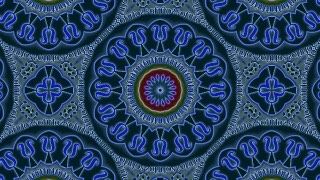• Twentyfold Mirrors 45 degrees :
20 rotations folding in a 45° lattice.
• Square & triangles Mirrors :
• Hexagons, squares & triangles mirror :
Semiregular tiling of Hexagons, triangles and squares.
• Decagons & pentagons mirror :
Semiregular tiling of decagons and pentagons.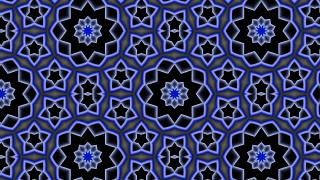• Pentagonal cristal mirror :
The pentagonal crystal mirror is especially interesting as tiling with regular pentagons is impossible. This algorithm offers a quasi crystal semiregular solution quite pleasing esthetically.##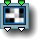22 Pixel prism

parameters :
A : Displacement (-4. : 4.)
B : Scale (-4. : 4.)
C : Frequency (-4. : 4.)

discussion :
Pixel Prism rearranges space by randomly breaking up the incoming space into little squares of uniform size. The effect is puzzle-like. To best visualize it, use the Movie or pict component after it as in the images below. With displacement at 0 and scale at 0, there is no re-arrangement of the incoming space though there may be tiling (depending on the setting of the frequency parameter which determines the size of the squares).

Warning: The output is highly discontinuous and is to be avoided in systems destined for ArtMatic Voyager.

Example: Pixel Prism with Displacement set high completely scrambles the geometry of XY space.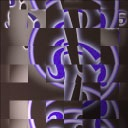##22 Crystallize

parameters :
A : Amplitude (0. : 1.)
B : Frequency (0. : 16.)
C : Phase (-16. : 16.)

discussion :
Deforms space in a painterly way that makes it great for creating stylization effects for video processing. The image below demonstrates its effect on the U&I Software logo.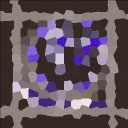Warning: The output is highly discontinuous and is to be avoided in systems destined for ArtMatic Voyager.

##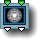22 Disk Tiling

parameters :
A : Smooth Edges (0. : 1.)
B : Offset x (-4. : 4.)
C : Offset y (-4. : 4.)

discussion :
Warp space with an Escher-like tiling of space into a disc-shaped enclosed space. The Tiling uses N Rotations folding with N increasing towards the edges. This is a great primitive for creating decorative figures.

##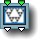22 Sierpinsky tiles

parameters :
A : Amplitude (0. : 8.)
B : Offset (-4. : 4.)
C : Frequency (0. : 8.)

discussion :
Sierpinsky tiles is a periodic implementation of a classic fractal algorithm that starts with a triangle and recursively fills space with smaller copies of itself in each triangles. The Tiling uses an hexagonal lattice with 3 alternating
Sierpinsky Triangles. This component is sensitive to the Maximum Iterations for Fractals preference.

##22 Ripples

parameters :
A : Frequency (-6.28 : 6.28)
B : Amplitude (-2. : 2.)
C : Phase (-32. : 32.)

discussion :
This component distorts space with concentric circles. 'Amplitude' provides the amount of distortion. With an amplitude of 0, there is no distortion. 'Frequency' determines the spacing of the ripples. For a realistic effect you can use the 'Phase' parameter to animate outward ripples.##22 Split & Move

parameters :
A : Angle (-180. : 180.)
B : Offset x (0. : 8.)
C : Offset y (0. : 8.)

discussion :
Splits the incoming space (plane) in two and moves each side in opposite directions. Infinity is generated in the space between the split planes so that the output of this component can be used as a mask for wipe effects.

##22 infinity disk gate

parameters :
A : Limit (0.25 : 64.)

discussion :
Modified in ArMatic engine 8.07, this component generates infinity values or remove infinities according to the following algorithms :

algorithms :

##22 Mirror

parameters :
A : Rotation (-180. : 180.)
B : Offset x (-32. : 32.)
C : Offset y (-32. : 32.)
D : Smoothness % (0. : 1.)

discussion :
Mirrors the input space along an orientable axis. The 'Rotation' parameter allows the axis of mirroring to be rotated which yields interesting patterns when this component is used in a looped tree. Options allows to set the angle in radians or degrees and existing values are remapped when changing the 'Rotation' unit.
Great fractals can be achieved with just mirroring and scaling. 'Smoothness' smooth the discontinuity at the mirroring line.
22 Mirror don't scale the space and is DF modeling friendly.

algorithms :
• Offset Output :
The 'Offset' parameters are applied after the mirroring offseting the mirrored space coordinates.
• Offset Mirror :
The 'Offset' parameters are applied prior the mirroring, thus offseting the mirror axis.

##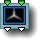22 Mirrors 3

parameters :
A : Scale (-4. : 4.)
B : Offset x (-8. : 8.)
C : Offset y (-8. : 8.)

discussion :
Mirror space into three parts with a 120 degree rotational symmetry. Use this and the other "mirror" components for kaleidoscopic effects. 'Scale' scales the incoming space and the offset parameters provide horizontal and vertical offsets of the resulting space. 'Offsets' are applied after the mirroring.

##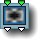22 Mirrors 4

parameters :
A : Scale (-4. : 4.)
B : Offset x (-8. : 8.)
C : Offset y (-8. : 8.)

##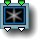22 N-Fold Mirrors #

parameters :
A : Scale (-4. : 4.)
B : Offset x (-50. : 50.)
C : Offset y (-50. : 50.)

discussion :
This component provides a number of different mirroring algorithms involving N rotational mirrors. Parameters options are available for all folding symmetry orientation options. Various algorithms basically sets the number of
rotational symmetries. 'Offsets' are applied after the mirroring. Keep 'Scale' at 1 for strict mirroring and DFRM compatibility.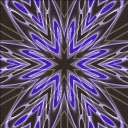algorithms :
• Skewed 6 Mirrors :
This algorithm was left for legacy and compatibility with older versions though it is mathematically incorrect. It creates a kaleidoscopic mirroring of space about three axes that are rotated 120 degrees apart.
• Threefold Mirrors :
• Fourfold Mirrors :
• Fivefold Mirrors :
• Sixfold Mirrors :
• Heightfold Mirrors :
• Ninefold Mirrors :
• Tenfold Mirrors :
• Twelvefold Mirrors :
• 45 degrees Mirrors :

##22 Branch N

parameters :
A : Branch number (1 : 50)
B : Offset x (-8. : 8.)
C : Offset y (-8. : 8.)
D : Smoothness % (0. : 8.)

discussion :
Branch N mirrors space as in the other "mirror" tiles but provides parametric control of the number of rotational folding mirrors as well as choices of mirror orientations. The 'Branch number' 'parameter allows you to achieve from 2 to 50
rotational symmetries. Unlike the other mirror functions, the axis symmetry can be made seamless (continuous) using the 'Smoothness' parameter which allows to create continuous surfaces. The 'offset x' and 'offset y' parameters are the horizontal and vertical offsets of the output mirrored space.

Parameter options provide a number of different symmetries. When inward is chosen, the orientation matches the one used by 3D Mirrors & Rotates # .

• Orient outward (x-,y-)
• Orient inward (x-,y+)
• Orient outward (x+,y-)
• Orient inward (x+,y+)

##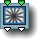22 Radialstar

parameters :
A : Outer phase (0. : 12.57)
B : Narrowness (0. : 1.)
C : Amplitude (0. : 8.)

discussion :
This component distorts space continuously with radial undulations centered around origin. 'Amplitude' controls amount of displacement. Animate the outer phase parameter for nice explosion effects.

##22 Disk Mirror

parameters :
A : Disk Size (0.25 : 50.)
B : Mirror concavity (0. : 1.)
C : Offset y (-8. : 8.)
D : Offset x (-8. : 8.)

discussion :
Disk Mirror provides a circular mirroring of the incoming space. This is another component that can be used to constrain space to prevent it from reaching infinity. The 'Disk Size' parameter controls the radius above which the space is mirrored. 'Offset' x and y controls the center of the mirroring disk.
When 'Mirror concavity' is close to 1 the mirror reflects the inside and values in-between will distort the space to blend between orientations. Keep it at zero for a strict circle inversion.##22 Distance Mirror

parameters :
A : Amplitude (0. : 2.)
B : Mirror distance (0. : 24.)

discussion :
This component inwardly mirrors distance beyond a certain radius and hence is another component that can be used for avoiding infinities. Unlike Disk mirror the inversion of space is made progressive and continuous, hence it is not strictly speaking a mirroring function. The space inside the distance given by 'Mirror distance' parameter is left unmodified.

##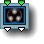22 Disk Inverse Ring

parameters :
A : Disk number (2. : 12.)
B : Scale (-8. : 8.)
C : Offset y (-1. : 1.)

discussion :
Mirrors the 2D space with a set of N tangents Mirroring circles arranged in a ring. 'Disk number' sets the number of disk mirrors while 'Scale' controls their radius and the underlying ring radius. When applied recursively disk inversions may creates fascinating fractals. 'Offset y' offsets the radius of an overall disk mirror that can go outward when positive or inward when negative. In general you will keep y at -1 (regions outside the overall disk mirror the inside), or 0,1 (regions inside the overall disk mirror the outside);

##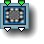22 Complex inverse

parameters :
A : Scale (0.03 : 2.)
B : Offset x (-32. : 32.)
C : Offset y (-32. : 32.)

discussion :
Complex inverse inverts space using the complex number division 1/Z, Z=(x+iy). The transformation divides (x,y) by its squared distance to zero so that infinity becomes (0,0) and (0,0) is mapped to infinity.
The Complex inversion has the remarkable property to transform lines into circles.
Complex Division.
Note : the euclidian distance or length(x,y) is also called the magnitude of the complex x+iy.

http://mathworld.wolfram.com/ComplexDivision.html

##22 Complex Moebius

parameters :
A : Angle (-6.28 : 6.28)
B : Scale (-4. : 4.)
C : Imaginary (-4. : 4.)

##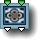22 Complex Map

parameters :
A : Real (-2. : 2.)
B : Imaginary (-2. : 2.)

discussion :
Complex Map transforms the 2D space with several iterations of the complex equation C(Z+1/Z) with C constant determined by the parameter A and B (C=A+iB). Z is set by the x,y inputs as the real and imaginary parts of a complex number : Z = x+iy.

##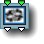22 Complex Map 2

parameters :
A : Real (-2. : 2.)
B : Imaginary (-2. : 2.)

discussion :
Complex Map 2 transforms the 2D space with several iterations of the complex polynomial ((Z^2 +C1) / (2Z + C2))^2. C1 and C2 are derived from the parameters A and B using C1=A-1+iB and C2=A-2+iB. This component treats the x,y inputs as a complex number Z with Z = x+iy.

##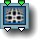22 Complex waves

parameters :
A : Scale (0. : 4.)
B : Offset (-2. : 2.)

discussion :
This component warps the incoming space by applying a low frequency sine wave transform.

##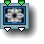22 Ring Space

parameters :
A : Offset x (-4. : 4.)
B : Offset y (-4. : 4.)
C : Warp (0. : 1.)

discussion :
This component warps space about several attractors. As with many of the complex number-based components, this component can be used to avoid infinities. The presence of the three attractors makes this component terrific for creating flower-like shapes.

##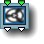22 Polar Space

parameters :
A : Polar scale (0.50 : 8.)
B : Distance scale (0.50 : 6.28)
C : Phase x (-3.14 : 3.14)

discussion :
Use this component to interpret x and y as polar coordinates where x is the angle and y is distance.

##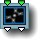22 Orbiters

parameters :
A : Rotation (-2. : 2.)
B : Offset (-2. : 2.)
C : Level (-2. : 2.)

discussion :
This is a complex component that re-arranges space into a set of hierarchical cells, each with its own coordinate system, rotating around the center larger cell. The 'Level' parameter controls the size of each cell while 'Rotation' animates the system and makes cells further apart. Space is blended between cells coordinates which creates a smooth distorted geometry.
The easiest way to see how this component re-arranges space is to follow it with the Movie/Pict component.
Example : Libraries/Components demo/22 Orbiter.artm

##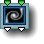22 Twirl

parameters :
A : Scale (0. : 1.)
B : Angle (-4. : 4.)

discussion :
Twirl distorts space with a whirlpool pattern.

##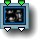22 Multi twirls

parameters :
A : Frequency (0.06 : 4.)
B : Phase (-4. : 4.)
C : Twirl (0. : 8.)

discussion :
Multi twirls distorts space with randomly placed whirlpools. This is a great component for distorting pictures especially when creating animation. This component may also be used to create storm structures on a planet atmosphere or ArtMatic clouds layers for ArtMatic Voyager.##22 Tech displace

parameters :
A : Displacement (0. : 4.)
B : Phase (-16. : 16.)
C : Frequency (0. : 4.)

discussion :
A displacement map with a sparse geometric texture. 'Displacement' controls the amount of distortion while 'Frequency' sets the displacement texture scale.

Example: A square grid displaced by "tech Displace".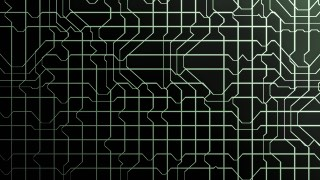##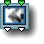22 Tech quantize

parameters :
A : Displacement (0. : 1.)
B : Phase (-16. : 16.)
C : Frequency (0. : 4.)

discussion :
"Tech quantize" uses a random arrangement of 45-degree orthogonal patterns to quantize the input, creating mosaic effects. Warning : The output space is highly discontinuous and is to be avoided for terrains & volumes design.##22 Random noise

parameters :
A : Amplitude (-2. : 2.)
B : Phase (-8. : 8.)
C : Frequency (-32. : 32.)

discussion :
This component provides continuous random distortions of the (x,y) space with two narrow band random noises. The distortion can be subtle or extreme depending on the 'Amplitude' value.

##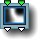22 Low Freq Random

parameters :
A : Amplitude (0. : 8.)
B : Phase (-8. : 8.)
C : Frequency (0. : 16.)

##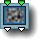22 Perlin random

parameters :
A : Amplitude (0. : 4.)
B : Phase (-32. : 32.)
C : Frequency (0. : 32.)

discussion :
A Perlin noise-based component that combines the output of two uncorrelated
Perlin Noise functions for each output to displace the input space. The 'Amplitude' parameter controls the amount of displacement of the incoming values. 'Phase' offsets the noise in x. 'Frequency' has the standard frequency options and controls the scale of the noise.

##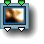22 Multi Perlin displace

parameters :
A : Amplitude (0. : 4.)
B : Octaves (1. : 128.)
C : Frequency (0. : 32.)
D : Roughness (0.12 : 0.75)

discussion :
To displace the input space, "Multi Perlin displace" uses two uncorrelated Multi-Perlin noises functions for each coordinates. The Multi-Perlin noise sums
Perlin Noise functions at various frequencies according to the 'Octaves' parameter.
The 'Amplitude' parameter controls the amount of displacement. 'Frequency' has the standard frequency options and controls the scale of the noise. 'Roughness' controls the fractal dimension and has a visible effect only when octaves is above 2.

##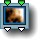22 2D turbulence

parameters :
A : Amplitude (-2. : 2.)
B : Phase (-32. : 32.)
C : Frequency (0. : 32.)
D : Roughness (0.25 : 0.65)

discussion :
This is a 2D fractal displacement function whose 'Amplitude' parameter controls the amount of displacement added to the input space. This component is great for creating turbulence, fire, marble and clouds. 2D Turbulence is based on two parallel fractal noises. See the notes for
"2D turbulence" is sensitive to the Max iterations for fractals preference, as most fractal noises/displacement functions. The 3D equivalent of this component can be found at 32 Turbulence 3D .
'Frequency' has the standard frequency options while 'Roughness' controls the fractal dimension.

##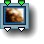22 Time turbulence

parameters :
A : Amplitude (0. : 8.)
B : feedback (-8. : 8.)
C : Frequency (0. : 32.)
D : Pace (0. : 6.28)

##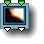22 MultiFractal displace

parameters :
A : Amplitude (0. : 2.)
B : Smoothness % (0. : 1.)
C : Frequency (0. : 32.)
D : Roughness (0.20 : 0.80)

discussion :
A 2D fractal displacement noise created by summing vector-valued Perlin noise functions of varying fractal dimension (roughness). "Multi-Fractal" describes fractals with varying fractal dimension (a measure of the surface roughness). See the scalar version of
MultiFractal noise.

Multi Fractal displace creates rich and natural textures - especially when used in ArtMatic Voyager. 'Amplitude' controls the amount of displacement of the input values. Smoothness controls the roughness of the displacement texture.
'Frequency' has the standard frequency options while 'Roughness' controls the fractal dimension.A Multi-Fractal displaced cone rendered in ArtMatic Voyager.

##22 Ridged Fractal displace

parameters :
A : Amplitude (0. : 2.)
B : Phase (-32. : 32.)
C : Frequency (0. : 32.)
D : Roughness (0.25 : 0.75)

discussion :
A multi-fractal 2D vector function based on a "ridged" version of the Perlin noise function as discussed in the 2D Scalar (21) components chapter. See
21 Ridged Fractal noise. 'Amplitude' controls the amount of displacement of the input space.
'Frequency' has the standard frequency options while 'Roughness' controls the fractal dimension.

##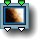22 Chaos displace A

parameters :
A : Amplitude (0. : 1.)
B : Feedback (-1. : 1.)
C : Frequency (0. : 32.)
D : Roughness (0.25 : 0.75)

discussion :
A composite multi-fractal displacement function based on a cubic-clipped Perlin noise function. 'Frequency' has the usual
frequency options settings while 'Roughness' controls the fractal dimension.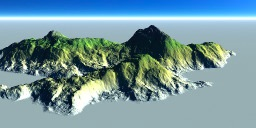Chaos displace can turn a simple cone into a rich featured island.

##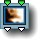22 Chaos displace B

parameters :
A : Amplitude (0. : 2.)
B : Feedback (-1. : 1.)
C : Frequency (0. : 32.)
D : Roughness (0.25 : 0.75)

discussion :
A composite multi-fractal displacement function based on an algorithm similar the
21 Bubble Rock component. As with Chaos displace A it can turn simple surface into highly complex fractal terrains.
'Frequency' has the usual frequency options settings.

##22 Crystal displace

parameters :
A : Amplitude (0. : 8.)
B : Phase (-16. : 16.)
C : Frequency (0. : 32.)
D : Roughness (0.25 : 0.75)

##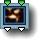22 Random Fractal Space

parameters :
A : Amplitude (0. : 32.)
B : Phase (-32. : 32.)
C : Frequency (0. : 32.)
D : Roughness (0.20 : 0.80)

##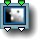22 Spots displace

parameters :
A : Displacement (0. : 8.)
B : Phase (-32. : 32.)
C : Frequency (0. : 8.)

discussion :
A displacement function comprised of randomly sized bumps placed in an infinite lattice. 'Displacement' controls the amount of space distortion while 'Frequency' and 'Phase' sets the underlying grid scale and translation in x.##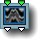22 Directional Random

parameters :
A : Amplitude (0. : 2.)
B : Direction (-2. : 2.)
C : Phase (-16. : 16.)

discussion :
This component distorts the incoming 2D space in a particular direction provided by the 'Direction' parameter. When direction is 2 or -2, space is distorted vertically. When it is set to 0, the distortion is purely horizontal. In-between values, provide skewed distortion. 'Amplitude' controls both amplitude and frequency.

##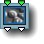22 Bubble Space

parameters :
A : Amplitude (0. : 2.)
B : Bubble size (1. : 6.)
C : Frequency (0. : 32.)

discussion :
As with the
22 Orbiters component, bubbles creates cells enclosing their own space : Each bubble has 0 at its center and is surrounded by 0. Cells are randomly placed and space is blended between cells or "Bubbles" so the resulting space is continuous while highly distorted. 'Bubble size' controls the size and overlapping of bubbles.
This component is terrific for creating non-repeating and organic looking textures and random patterns.
'Frequency' has the standard frequency options settings.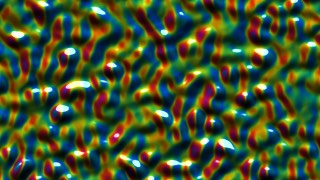##22 Facet space

parameters :
A : Scale (0. : 2.)
B : Delta size (0. : 6.)
C : Frequency (0. : 8.)

##22 Facet space mirrors

parameters :
A : Scale (0.12 : 2.)
B : Delta size (0. : 6.)
C : Frequency (0. : 8.)

discussion :
Similar to "Facet space" this component creates random facets/tiles using Voronoi diagrams but uses symmetrical space for each cells. The transitions between the facets is smooth which makes it a great tool for creating natural semi-random textures. 'Scale' affects the size of coordinates within each facet while 'Frequency' sets the period of the facet non periodic texture. 'Delta size' adjust how much facets coordinates are randomized in scale.Facet space mirrors shaded with U & I logo

##22 Facet space rotate

parameters :
A : Delta angle (0. : 6.28)
B : Delta size (0. : 6.)
C : Frequency (0. : 8.)

discussion :
Similar to the Facet Space component but each cell is randomly rotated. The "Delta Angle" parameter controls the amount of random rotation.

## 22 Compiled Tree :

parameters :
A : Blend (0:1)
B : Recursions

discussion :
Compiled trees are groups of tiles that can be used in place of single tiles as a kind of macro or subroutine. 22 CTs can hold groups of 2D space transformations or can be used to manipulate 2 parallel streams of datas : scalar single values (1D), 3D or 4D packed vectors.

usage :
Select a 22 tile and use "New compiled tree" to create a new CT from the selection (Tree Edit menu or type 'n' key).
To save a CT on disk to use the function elsewhere use "Save compiled tree" from the Tree Edit menu.
You may also copy and paste the entire CT by using Copy Tile and Paste Tile from the Edit menu.

22 CT can be used recursively if the option "Allow feedback" is set. In that case output is fed to the input at second iteration and the transform will be applied N times, N being set by the "Recursions" parameter. Recursions over 2D space transforms provide a simple and efficient way to generate 2D fractals.
22 CT allows recursion with feedback ON with packed RGBA streams as well as scalar input/outputs. The types of ins and outs has to match for the feedback to function properly. If ins and outs are packed RGBA, a 22 CT can process 8 values. This vector usage of 22 CT is often needed for plant design where each level has to send both transformed space coordinates and textured DF RGBA data to the next. Examples can be found in Voyager Library/3D Models/DF Plants folder.